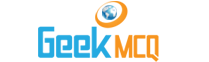# Engineering :: Thermodynamics

41.  In the polytropic process pvn = Constant if n = 1 the process is termed as
 A. Constant volume B. Constant pressure C. Constant temperature D. Adiabatic E. Isothermal

42.  A law which is applicable only to ideal vapours and liquids, that equates the equilibrium partial pressures of a solution component in the coexisting phases, is known as
 A. Henry's law B. Roult's law C. Joule's law D. Maxwell's equation E. Fick's law

43.  The molecular volume of any perfect gas at 600 x 103 N/m3 and 27?C will be
 A. 2.085 m3/kg mol B. 4.17 m3/kg mol C. 41.7 m3/kg mol D. 85.3 m3/kg mol E. 47.7 m3/kg mol

44.  An open system
 A. Is a specified region where transfers of energy and/or mass take place B. Is a region of constant mass and only energy is allowed to cross the boundaries C. Cannot transfer either energy or mass to or from the surroundings D. Has a mass transfer across its boundaries, and the mass within the system is not necessarily constant E. None of the above

45.  A system consisting of more than one phase is known as
 A. Open system B. Closed system C. Isolated system D. Non-uniform system E. Heterogeneous system

46.  Whenever a system undergoes either a change in state or an energy or mass transfer at a steady state, it is said to undergo
 A. A change of state B. A process C. A steady state transfer D. An equilibrium E. A thermodynamic change

47.  The processes or systems that do not involve heat are called
 A. Isothermal processes B. Equilibrium processes C. Thermal processes D. Steady processes E. Adiabatic processes

48.  Second law of theromodynamics defines
 A. Heat B. Work C. Enthalpy D. Entropy E. Internal energy

49.  In a thermal power plant thermodynamic cycle used is
 A. Erricson B. Brayton C. Joule D. Carnot E. Rankine

50.  For the same heat inpur and same compression ratio
 A. Otto cycle and Diesel cycle are equally efficient B. Otto cycle is less efficient than diesel cycle C. Efficiency depends mainly on working substance D. None of the above